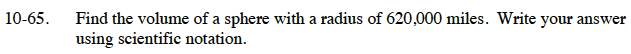Home > CC3 > Chapter Ch10 > Lesson 10.1.5 > Problem10-65

10-65.

Find the volume of a sphere with a radius of 620,000 miles. Write your answer using scientific notation. Homework Help ✎$\text{The formula to find the volume of a sphere is {\it V}=\frac{4}{3}\pi{\it r}^3.}$

When you write a number in scientific notation, the number that is multiplied by a power of 10 should be between 1 and 10.

9.98 × 1017